# Class 12 Photoelectric Effect

4517

Physics Modern Physics Level: Misc Level

Imagine a photon strikes a metal plate that has a work function of Theta =3 x 10 to the - 19 power J. If the photon has an energy of 3.4 x 10 to the negative 18 power J, what is the maximum kinetic energy of the ejected electrons?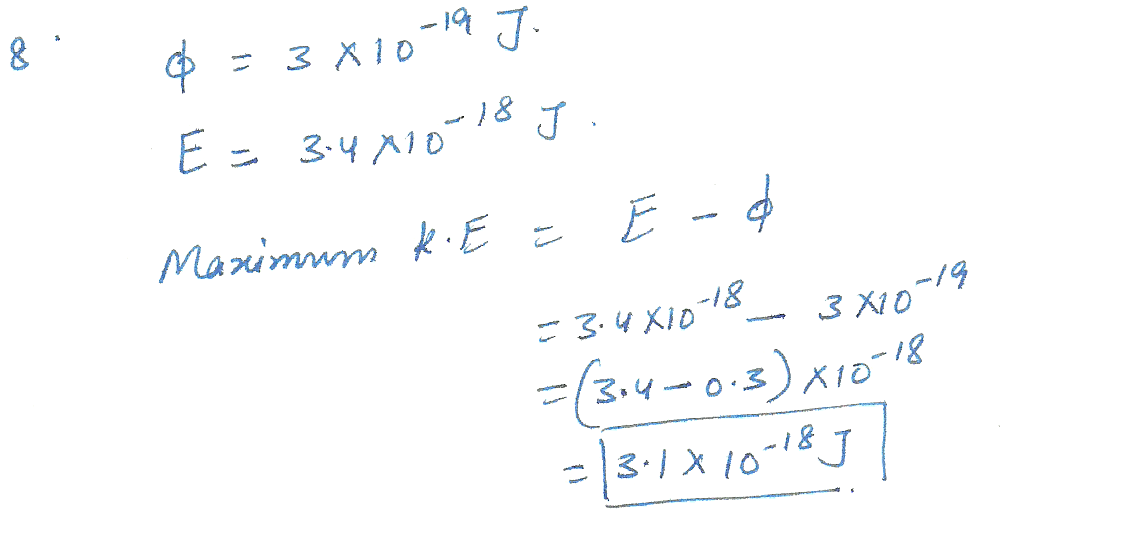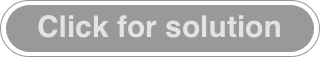4126

Physics Modern Physics Level: Misc Level

The photoelectric threshold wavelength for pure sodium metal is 546 nm. How much energy (in electronvolts ) is needed to tear an electron away from this metal?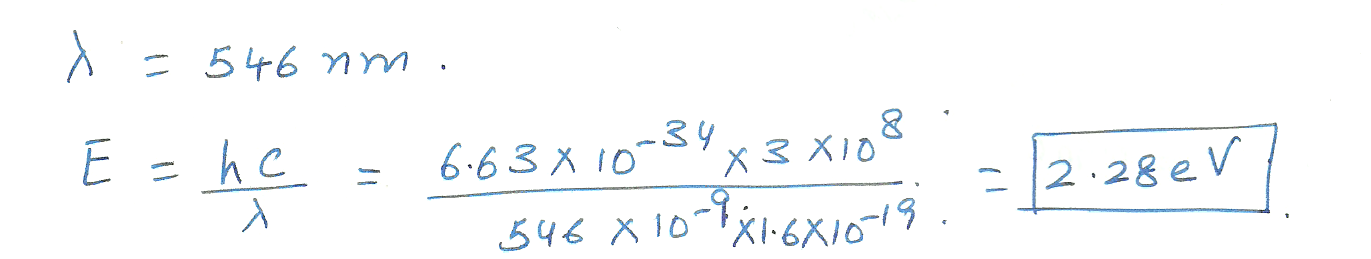3531

Physics Modern Physics Level: University

Dual nature Photo electric effect

The minimum energy required to remove an electron from a metal is 2.60 eV.What is the longest wavelength photon that can eject an electron from this metal?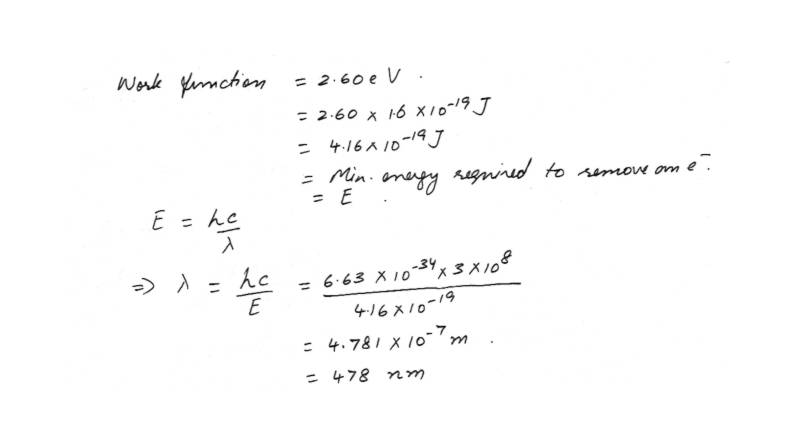3008

Physics Modern Physics Level: High School

Photoelectric Effect

A magnesium surface has a work function of 3.78 eV . Electromagnetic waves with a wavelength of 215 nm strikes the surface and eject electrons . Find the maximum kinetic energy of the ejected electrons . Express your answer in electron volts .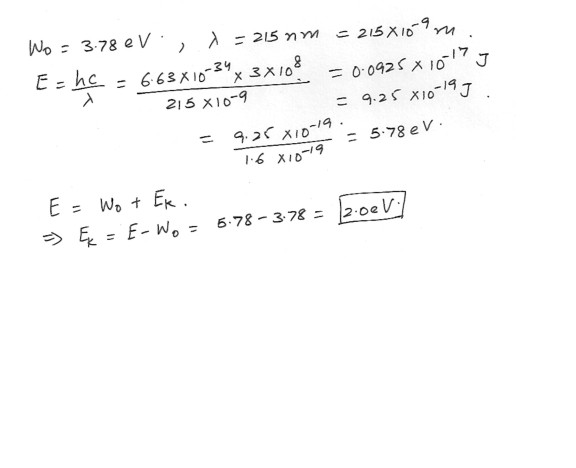3007

Physics Modern Physics Level: High School

Photoelectric Effect

Ultraviolet light with a frequency of 2.50 1015 Hz strikes a metal surface and ejects electrons that have a maximum kinetic energy of 6.9 eV . What is the work function (in eV) of the metal ?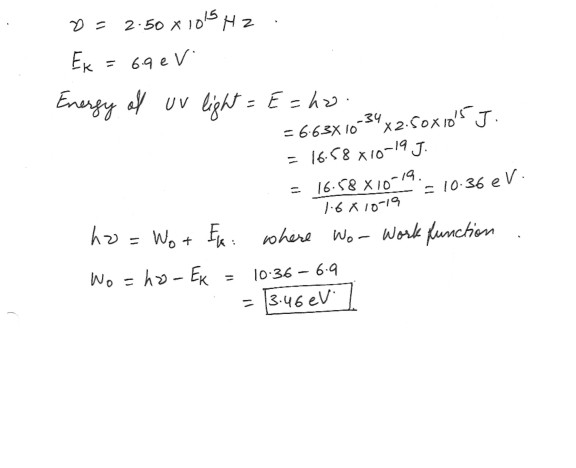2583

Physics Modern Physics Level: High School

Photoelectric Effect

The photoelectric effect is important for two reasons , firstly , it provides strong evidence for the particle nature of light , and secondly , it has a large number of practical applications .

a) Give one experimental observation coming from the study of the photoelectric effect that provides strong support for the particle theory of light .

b) State two practical applications of the photoelectric effect .

c) The work function of sodium is 2.46 eV .

i) Find the maximum frequency of radiation needed to eject photoelectrons from a sodium surface .

ii) If the sodium , surface is illuminated with radiation of wavelength 3.00 x 10^-7 metres , calculate the maximum kinetic energy of ejected photoelectrons .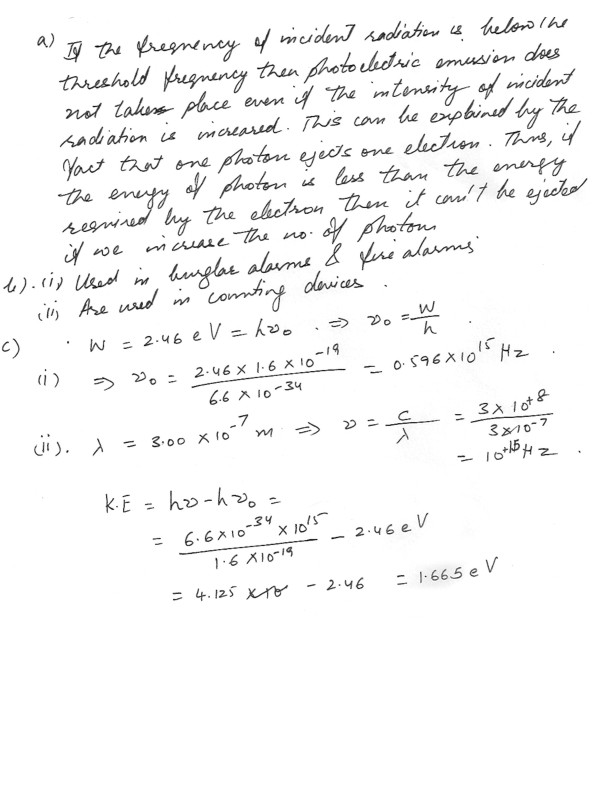2469

Physics Modern Physics Level: High School

Photoelectric Effect

A Light of frequency 9.0 x 10^14 Hz incident on a metal surface is ejects photo-electrons having energy 1 eV . Calculate the work function W for this metal .2454

Physics Modern Physics Level: High School

Photoelectric Effect

Radiation with a wavelength of 238 nm shines on a metal surface and ejects electrons that have a maximum speed of 3.75 x 10^5 m/ s . Which one of the following metals is it , the values in parentheses being the work functions : potassium (2.24 eV) , calcium (2.71 eV) , uranium (3.63 eV), aluminum(4.08 eV) , or gold (4.82 eV) .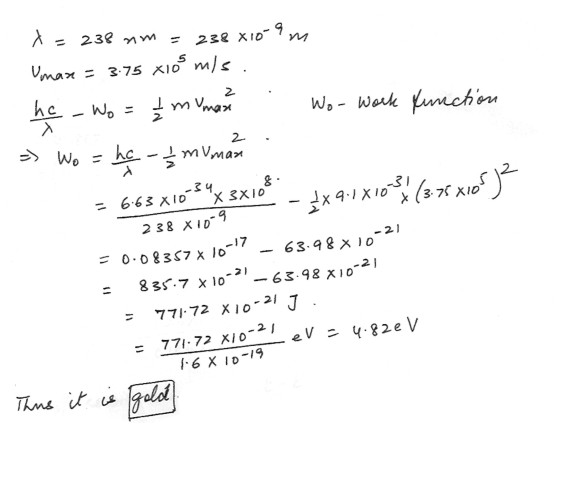2449

Physics Modern Physics Level: High School

Dual Nature

Gold has a work function W of 4.9 eV .

a) What is the minimum frequency of photon needed to observe the photoelectric effect ?

b) If illuminated by ultraviolet radiation of frequency 2.3 x 10^15 Hz what is the K.E in Joules of the emitted electron ?

c) What is the energy in electron volts ?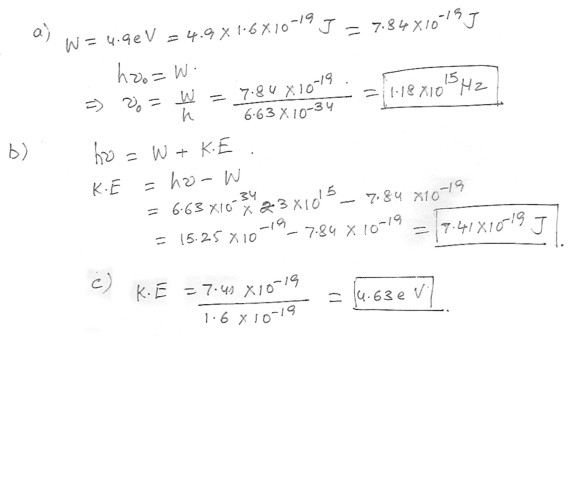2445

Physics Modern Physics Level: High School

Photoelectric Effect

The work function for cesium is 2.0 eV . Find the maximum speed of the ejected electrons when a cesium surface is illuminated by violet light of wavelength 4.5 x 10 ( to the -7) m .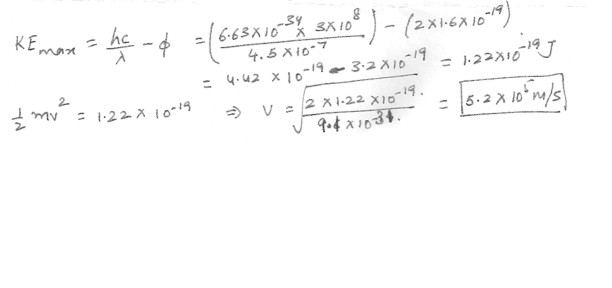Displaying 1-10 of 12 results.